## Functions of Acute Angles

The characteristics of similar triangles, originally formulated by Euclid, are the building blocks of trigonometry. Euclid's theorems state if two angles of one triangle have the same measure as two angles of another triangle, then the two triangles are similar. Also, in similar triangles, angle measure and ratios of corresponding sides are preserved. Because all right triangles contain a 90° angle, all right triangles that contain another angle of equal measure must be similar. Therefore, the ratio of the corresponding sides of these triangles must be equal in value. These relationships lead to the trigonometric ratios. Lowercase Greek letters are usually used to name angle measures. It doesn't matter which letter is used, but two that are used quite often are alpha (α) and theta (θ).

Angles can be measured in one of two units: degrees or radians. The relationship between these two measures may be expressed as follows:The following ratios are defined using a circle with the equation x 2 + y 2 = r 2 and refer to Figure 1 .Figure 1
Reference triangles.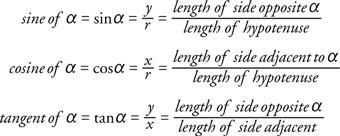Remember, if the angles of a triangle remain the same, but the sides increase or decrease in length proportionally, these ratios remain the same. Therefore, trigonometric ratios in right triangles are dependent only on the size of the angles, not on the lengths of the sides.

The cosecant, secant, and cotangent are trigonometric functions that are the reciprocals of the sine, cosine, and tangent, respectively.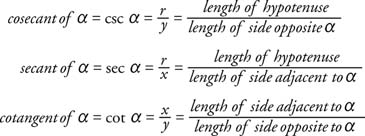If trigonometric functions of an angle θ are combined in an equation and the equation is valid for all values of θ, then the equation is known as a trigonometric identity. Using the trigonometric ratios shown in the preceding equation, the following trigonometric identities can be constructed.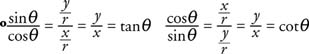Symbolically, (sin α) 2 and sin 2 α can be used interchangeably. From Figure (a) and the Pythagorean theorem, x 2 + y 2 = r 2.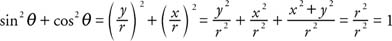These three trigonometric identities are extremely important: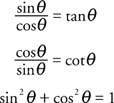Example 1: Find sin θ and tan θ if θ is an acute angle (0° ≤ θ ≤ 90°) and cos θ = ¼.Example 2: Find sin θ and cos θ if θ is an acute angle (0° ≤ θ ≤ 90°) tan θ = 6.

If the tangent of an angle is 6, then the ratio of the side opposite the angle and the side adjacent to the angle is 6. Because all right triangles with this ratio are similar, the hypotenuse can be found by choosing 1 and 6 as the values of the two legs of the right triangle and then applying the Pythagorean theorem.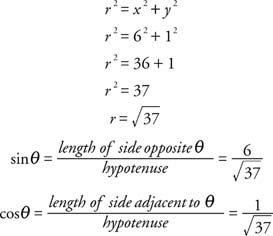Trigonometric functions come in three pairs that are referred to as cofunctions. The sine and cosine are cofunctions. The tangent and cotangent are cofunctions. The secant and cosecant are cofunctions. From right triangle XYZ, the following identities can be derived:Using Figure 2 , observe that ∠X and ∠Y are complementary.Figure 2
Reference triangles.

Thus, in general: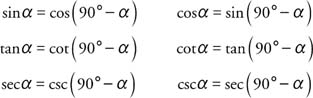Example 3: What are the values of the six trigonometric functions for angles that measure 30°, 45°, and 60° (see Figure 3 and Table 1 ).

 TABLE 1 Trigonometric Ratios for 30°, 45°, and 60° AnglesFigure 3
Drawings for Example 3
.